### Home > CC1 > Chapter Ch8 > Lesson 8.3.1 > Problem8-93

8-93.
1. Calculate each of the following quotients. Homework Help ✎

1.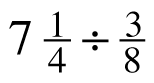2. 9.82 ÷ 0.2

3.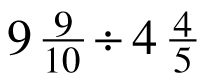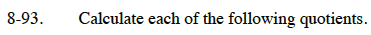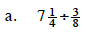Begin by rewriting the mixed number as a fraction greater than one.

$7\frac{1}{4}\div\frac{3}{8}=\frac{29}{4}\div\frac{3}{8}$

Calculate the quotient by multiplying by the multiplicative inverse.

$\frac{29}{4}\div\frac{3}{8} = \frac{29}{4}\cdot\frac{8}{3}$

Carry out the multiplication and then reduce the fraction to its simplified form.

$\frac{58}{3} = 19\frac{1}{3}$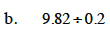Solve through regular long division. Don't forget to count the decimal places and move the decimal point in the quotient!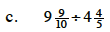Follow the process outlined in part (a).